# Table functions for transforming subtables

All the C# code examples presented on this page can be found in the related demo function of MS Visual Studio project FinaquantProtosStarter which can be downloaded at the product page of the free .NET library finaquant® protos.
Sometimes, you want to execute the same set of table operations (table function) on every subtable of an input table; this is a quite common calculation pattern in many applications in business and science.

Subtable transformation1 is a simple way of applying the same table function on every homogenous subtable of an input table.

Following scenarios may give an idea about the use of subtable transformation:

• You have a complex formula for computing the expected population of a city depending on historical data like birth rate, migration, population and so on. You have a table containing all these historical data for many cities, and you want to apply the same formula on the sub-data (i.e. subtable) of each city to obtain the expected population for each city.
• You have a well-tested standard function for calculating the performance of a financial asset depending on parameters like historical prices and benchmark values. You have a table containing all these parameters for multiple assets, and you want the obtain the performance measure for every asset in the table.
• You have a quite complex matrix function written with another library for matrix computations like ILNumerics, R or matlab, which calculates some statistical estimations based on historical data related with an entity like city, person or product. You have an input table with historical data of multiple entities, and you want to apply your matrix function on sub-data (subtable) of each entity in order to obtain a result table comprising the estimations for each entity (see 3. example below: submatrix transformation).

## Expected average temperature in May

As an introduction to the code examples below, assume you have a table function for calculating the expected average temperature of a certain location next year in May, based on historical average temperatures.You have a table including historical average temperatures of many locations, and you want to calculate the expected temperature for each location. Then you need a subtable transformer like the table function TransformSubTables in the uncommercial .net library finaquant® protos.### Integration with other matrix libraries

If all the subtable fields (input and output) are numeric fields, subtables can also be fed to a matrix function instead of a table function (see submatrix transformer TransformSubMatrices() in the 3rd example below).

This transformation structure opens the door for integrating the table function library finaquant protos® with the libraries for matrix computations like ILNumerics, R and matlab, as the user-defined matrix function written in a .net language can be used just as an envelope for a matrix function written with the methods of another library. Look for upcoming articles at finaquant.com about this integration issue.

## Examples

Following table with historical monthly average temperatures and population will be used for all of the examples below as the primary input.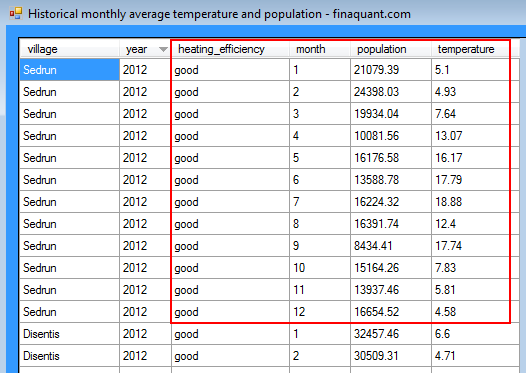This input table contains average monthly temperatures for the years from 2001 to 2012. The problem at hand is calculating expected energy consumption for each village and year in mega-joules.2 You may download this table as an excel file for a closer check:

The expected energy consumption for each month is given with the following formula:

`ExpectedEnergyConsumption = Population ÷ (50 + Temperature) x EfficiencyMultiplier`

… where EfficiencyMultiplier is given by the following parameter table for each category of heating efficiency: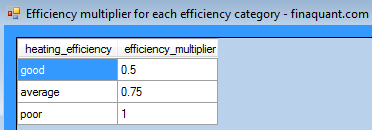Note that four fields are relevant for the calculation of expected energy consumption according to the given formula above: heating_efficiency, month, population and temperature.

These are the subtable input fields defining the frame of subtables that are to be fed to the table function for calculating the expected energy consumption. The first subtable corresponding to the remainder fields Sedrun-2012 is marked with a red-lined rectangle in the picture above showing the primary input table.

Even though the field month is not directly involved in the formula, it is a subtable field nevertheless, because the monthly consumption estimations must be summed up to obtain the yearly consumptions.

### Example 1: Testing user-defined table function ExpectedEnergyConsumption() with a single subtable (Sedrun, 2012)

Before calculating expected yearly energy consumptions for every subtable, let’s first test the user-defined table function ExpectedEnergyConsumption() as given below with a single subtable corresponding to remainder fields Sedrun-2012.

```// User-defined table function // Calculate expected yearly energy consumption (Mega-Joules) for heating // For each month: // Expected energy consumption = population / (50 + temperature) x EfficiencyMultiplier public static MatrixTable ExpectedEnergyConsumption(MatrixTable TemperatureSubTbl, params Object[] Parameters) { // PARAMETER CHECKS // table function for demonstration purposes, no elaborate parameter checks; // assumes parameters are entered correctly. MatrixTable EfficiencyMultTbl = (MatrixTable)Parameters; // combine input tables to have all parameters in a single table MatrixTable CombinedTbl = MatrixTable.CombineTablesFirstMatch(TemperatureSubTbl, EfficiencyMultTbl);   double Population, Temperature, Efficiency_Multiplier, Energy_Consumption = 0.0; TableRow trow;   for (int i = 0; i < CombinedTbl.RowCount; i++) { trow = CombinedTbl.GetTableRow(i);   // get parameters Population = TableRow.GetKeyFigureValue(trow, "population"); Temperature = TableRow.GetKeyFigureValue(trow, "temperature"); Efficiency_Multiplier = TableRow.GetKeyFigureValue(trow, "efficiency_multiplier");   // calculate energy consumption Energy_Consumption += Population / (50 + Temperature) * Efficiency_Multiplier; } // Return table with single key figure "energy_consumption" var EnergyConsumptionFields = TableFields.CreateEmptyTableFields(CombinedTbl.metaData); TableFields.AddNewField(EnergyConsumptionFields, "energy_consumption");   return MatrixTable.CreateTableWithElements_A(EnergyConsumptionFields, Energy_Consumption); }```

The rest is obtaining the subtable and feeding it to the table function shown above.

```// filter TemperatureTable with a condition table to obtain a subtable var CondTblFields = TableFields.CreateEmptyTableFields(md); TableFields.AddNewField(CondTblFields, "village"); TableFields.AddNewField(CondTblFields, "year"); // create condition table var CondTbl = MatrixTable.CreateTableWithElements_A(CondTblFields, "Sedrun", 2012 ); var SubTable = MatrixTable.FilterTableA(TemperatureTable, CondTbl); // column partition SubTable: exclude fields village and year SubTable = MatrixTable.ExcludeColumns(SubTable, TextVector.CreateVectorWithElements("village", "year")); // view subtable MatrixTable.View_MatrixTable(SubTable, "Subtable of TemperatureTable (Sedrun, 2012)");```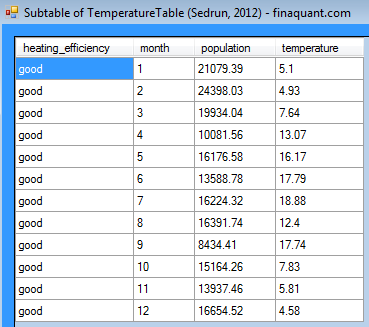```// execute user-defined table function to calculate expected energy consumption (Sedrun, 2012) MatrixTable EnergyConsumptionTbl = UserFunctions.ExpectedEnergyConsumption(SubTable, EffMultiplierTbl); // view result table MatrixTable.View_MatrixTable(EnergyConsumptionTbl, "CASE 1: Expected Energy Consumption (Sedrun, 2012)");```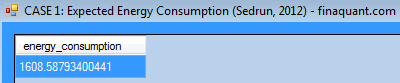This first example illustrates that the table function for calculating expected energy consumption works alright. We can now apply it on all subtables using the subtable transformer TransformSubTables().

### Example 2: Calculate expected energy consumption for each village and year

This example shows the power of subtable transformation. The table function ExpectedEnergyConsumption() tested in the previous example is now applied on every subtable of the primary input table.

```// CASE 2: Show the power of subtable transformation: // Calculate expected energy consumption for each village and year   // define subtable fields var SubTblFields = TableFields.CreateEmptyTableFields(md); TableFields.AddNewField(SubTblFields, "heating_efficiency"); TableFields.AddNewField(SubTblFields, "month"); TableFields.AddNewField(SubTblFields, "population"); TableFields.AddNewField(SubTblFields, "temperature"); // execute subtable transformer MatrixTable ResultTbl = MatrixTable.TransformSubTables(TemperatureTable, SubTblFields, UserFunctions.ExpectedEnergyConsumption, EffMultiplierTbl); // round all key figures to 2 digits after decimal point ResultTbl = MatrixTable.TransformKeyFigures(ResultTbl, x => Math.Round(x, 2), InputKeyFig: null, OutputKeyFig: null); // view result table MatrixTable.View_MatrixTable(ResultTbl, "CASE 2: Expected Energy Consumption for each village and year");```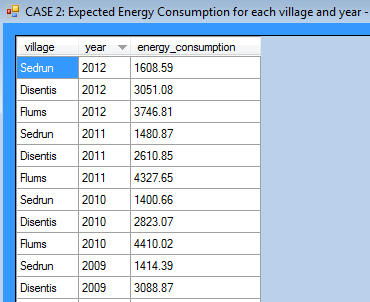### Example 3: Calculate expected energy consumption with submatrix transformation

In this example, the string category value heating_efficiency is first replaced by the numeric field efficiency_multiplier so that all relevant fields (i.e. subtable fields) for the calculation of expected energy consumption are numbers. These numeric subtables are then fed to user-defined matrix function ExpectedEnergyConsumptionMatrix() as matrix input through submatrix transformer TransformSubMatrices().

```// CASE 3: Calculate expected energy consumption with submatrix transformation   // prepare TemperatureTable for submatrix transformation // replace text attribute "heating_efficiency" with key figure "efficiency_multiplier" MatrixTable TempTbl = MatrixTable.CombineTablesFirstMatch(TemperatureTable, EffMultiplierTbl); // exclude field "heating_efficiency" TempTbl = MatrixTable.ExcludeColumns(TempTbl, TextVector.CreateVectorWithElements("heating_efficiency")); // view input table before submatrix transformation MatrixTable.View_MatrixTable(TempTbl, "Input table before submatrix transformation");``````// numeric attributes and key figures of submatrices that are to be transformed by // user defined matrix function UserFunctions.ExpectedEnergyConsumptionMatrix   // input fields as matrix columns TextVector NumAttributesIn = TextVector.CreateVectorWithElements("month"); TextVector KeyFiguresIn = TextVector.CreateVectorWithElements("population", "temperature", "efficiency_multiplier"); // output fields as matrix columns TextVector NumAttributesOut = TextVector.CreateEmptyVector(); TextVector KeyFiguresOut = TextVector.CreateVectorWithElements("energy_consumption"); // execute subtable transformation ResultTbl = MatrixTable.TransformSubMatrices(TempTbl, NumAttributesIn, KeyFiguresIn, NumAttributesOut, KeyFiguresOut, UserFunctions.ExpectedEnergyConsumptionMatrix); // round all key figures to 2 digits after decimal point ResultTbl = MatrixTable.TransformKeyFigures(ResultTbl, x => Math.Round(x, 2), InputKeyFig: null, OutputKeyFig: null); // view result table MatrixTable.View_MatrixTable(ResultTbl, "CASE 3: Expected Energy Consumption for each village and year");```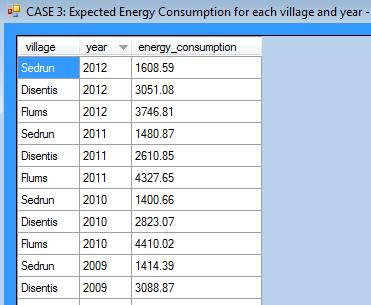You may compare the result table above with the results of the 2. example.
Written by: Tunç Ali Kütükçüoglu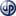Copyright secured by Digiprove © 2013 Tunc Ali Kütükcüoglu
1. The term “subtable transformation” is first used by the author of this article in this context and meaning. []
2. All the numbers and formulas used here for demonstration purposes are totally hypothetical without any relation to reality. []
This entry was posted in Calculation engine and tagged , , . Bookmark the permalink.